Call Us 1-631-753-8390

# Briefs Sizing Chart

Measuring for the Correct Size Brief

1. Measure the hips or waist, whatever is larger. Measure hipbone to hipbone and over the stomach.
2. After obtaining the hipbone to hipbone measurement, double the measurement and add two inches.

Example :
Hipbone to hipbone = 22"
22" X 2 = 44" + 2" = 46"
46" measures into a large brief

 Use the Height Conversion Chart below to find your height in inches 59" = 4'11" 60" = 5'0" 61" = 5'1" 62" = 5'2" 63" = 5'3" 64" = 5'4" 65" = 5'5" 66" = 5'6" 67" = 5'7" 68" = 5'8" 69" = 5'9" 70" = 5'10" 71" = 5'11" 72" = 6'0" 73" = 6'1" 74" = 6'2" 75" = 6'3" 76" = 6'4" 77" = 6'5" 78" = 6'6"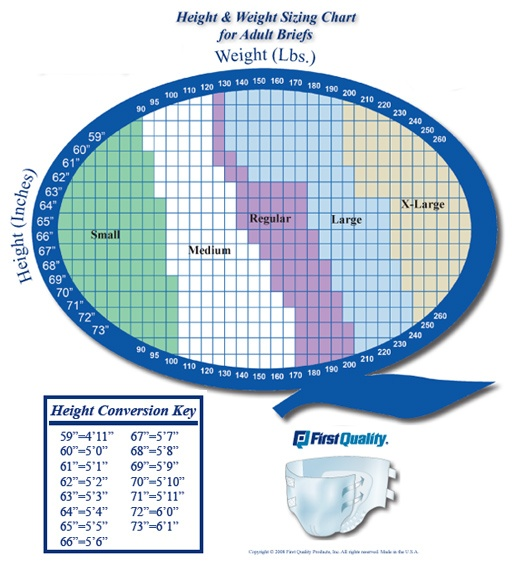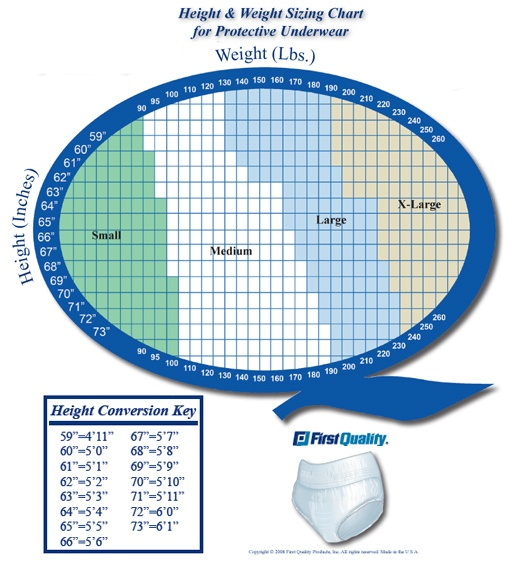Absorbency Key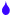Light Protection -   Symptom: Sudden, involuntary voiding of small amounts of urine.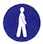Moderate Protection-   Symptom: Sudden, involuntary voiding of small to moderate amounts of urine.Heavy Protection-   Symptom: Uncontrolled daily voiding of moderate to large amounts of urine and/or stool.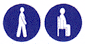Maximum Protection-   Symptom: Uncontrolled daily voiding of large amounts of urine and/or stool.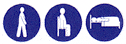Ultimate Protection -   Total loss of bladder and bowel control.